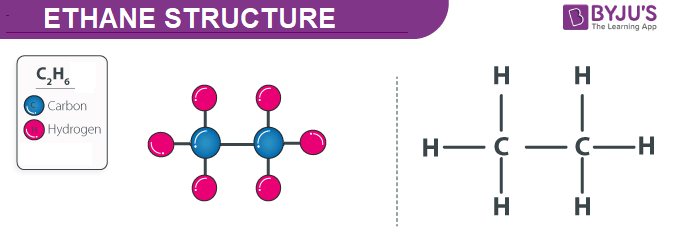# Rajasthan Board Question Paper for Class 10th General Science 2015 In PDF

## Rajasthan 10th Standard General Science Exam Question Paper 2015 with Solutions – Free Download

Rajasthan Board Class 10 General Science 2015 Question Paper and Solutions are useful for the students who prepare for the board exams. These solutions lay the basis for the Science exam. Questions asked in these RBSE Class 10 Science 2015 Question Paper solutions cover almost all the key concepts and topics from the textbook of the specific year, thus helping students to prepare well for exams.

The solved or unsolved question paper PDFs, can be accessed by the students by clicking on the respective links mentioned below, in this article. These interactive PDF links listed below allow the students to download the printable formats of the question papers. Meanwhile, the questions and answers are also published on the webpage.

Meanwhile, the students are urged to familiarise themselves with the complex topics from the RBSE Class 10 General Science textbook. Practising these solutions is the best way for the students to get an overview of the RBSE Class 10 General Science exam question paper pattern. Students are advised to solve the previous papers of Rajasthan Board Class 10 General Science while preparing for the exams. Solving these papers and referring to the solutions will most likely help the students to gauge their performance and self analyse their preparation level for the final exams. If the students solve these paper solutions regularly, they can get information about the marks’ distribution in each section.

### Rajasthan Board Class 10 General Science 2015 Question Paper with Solutions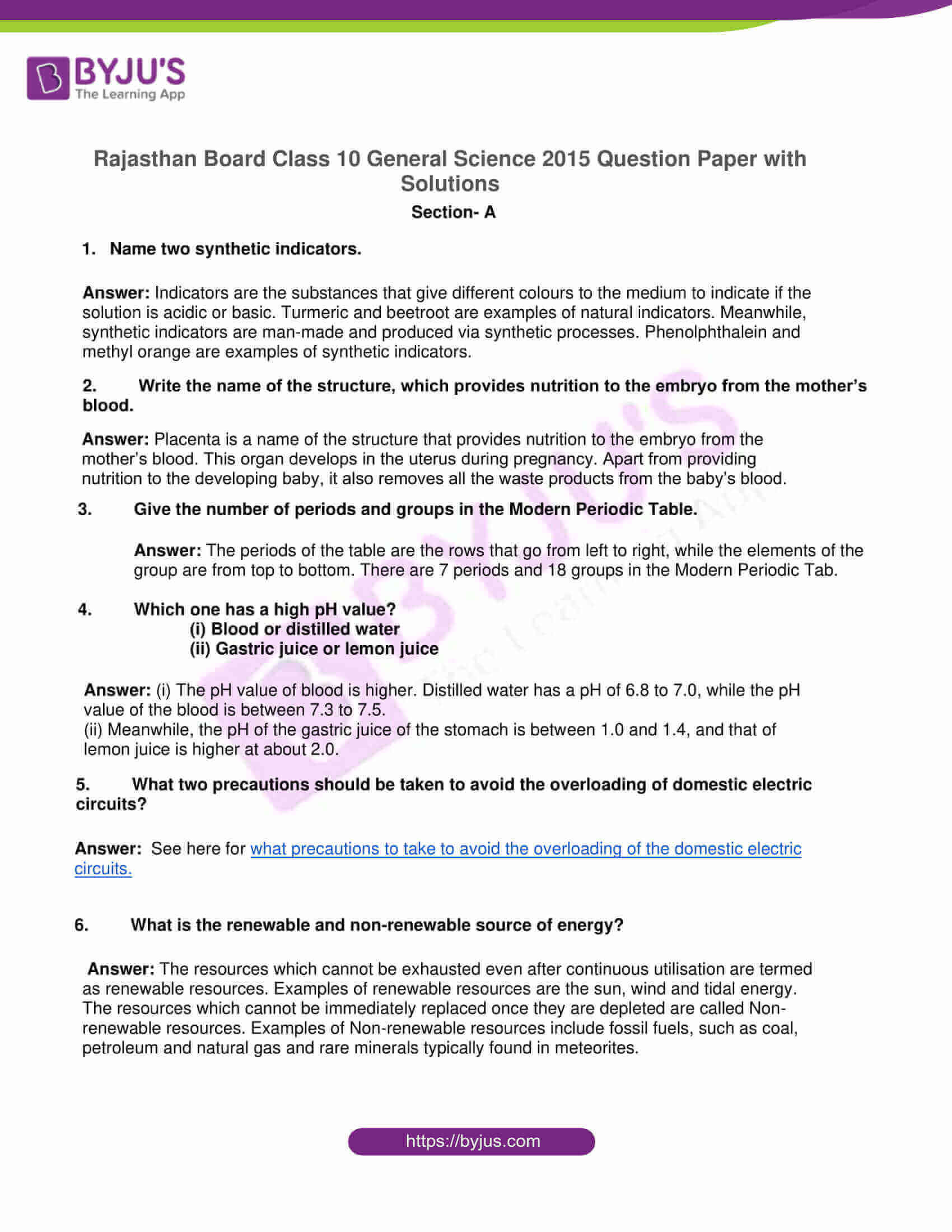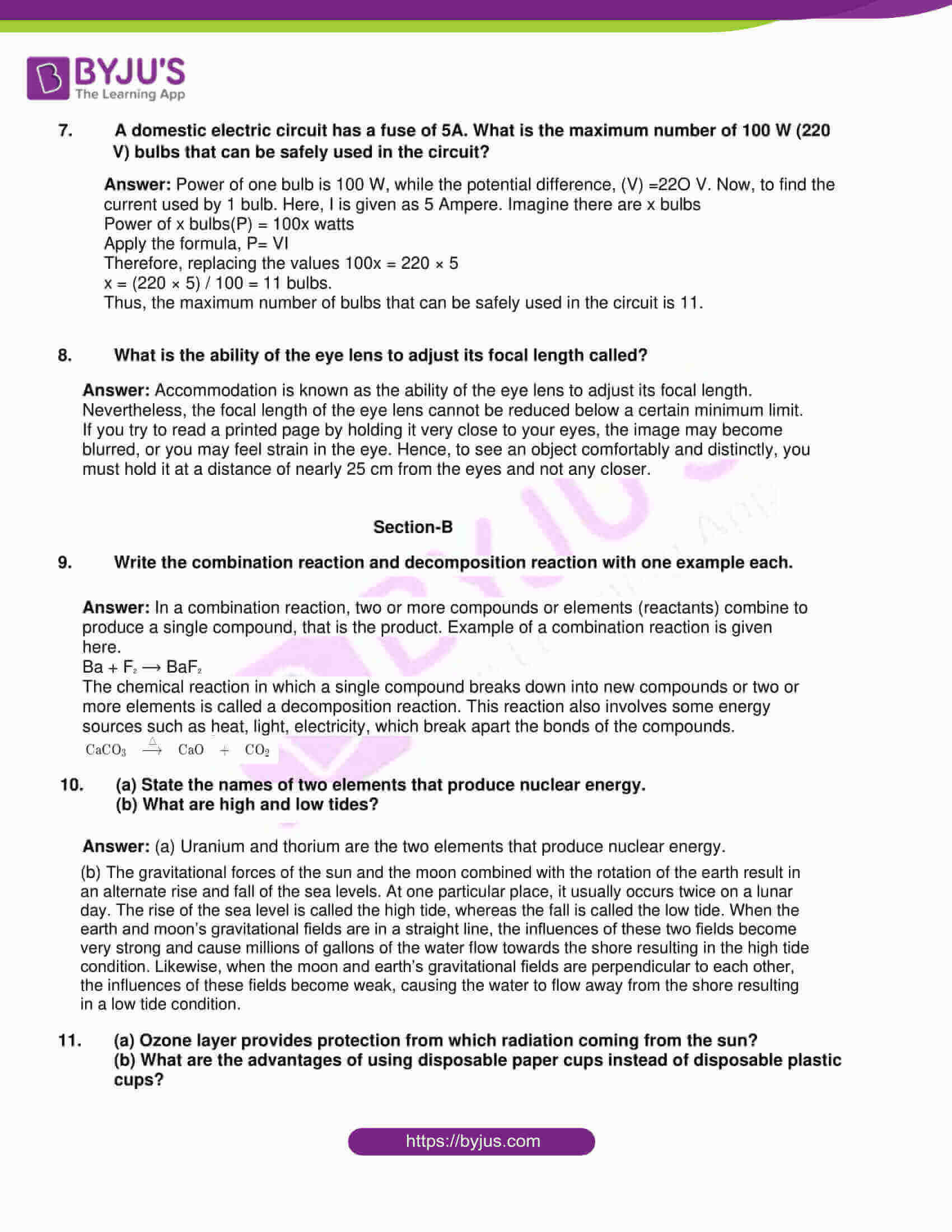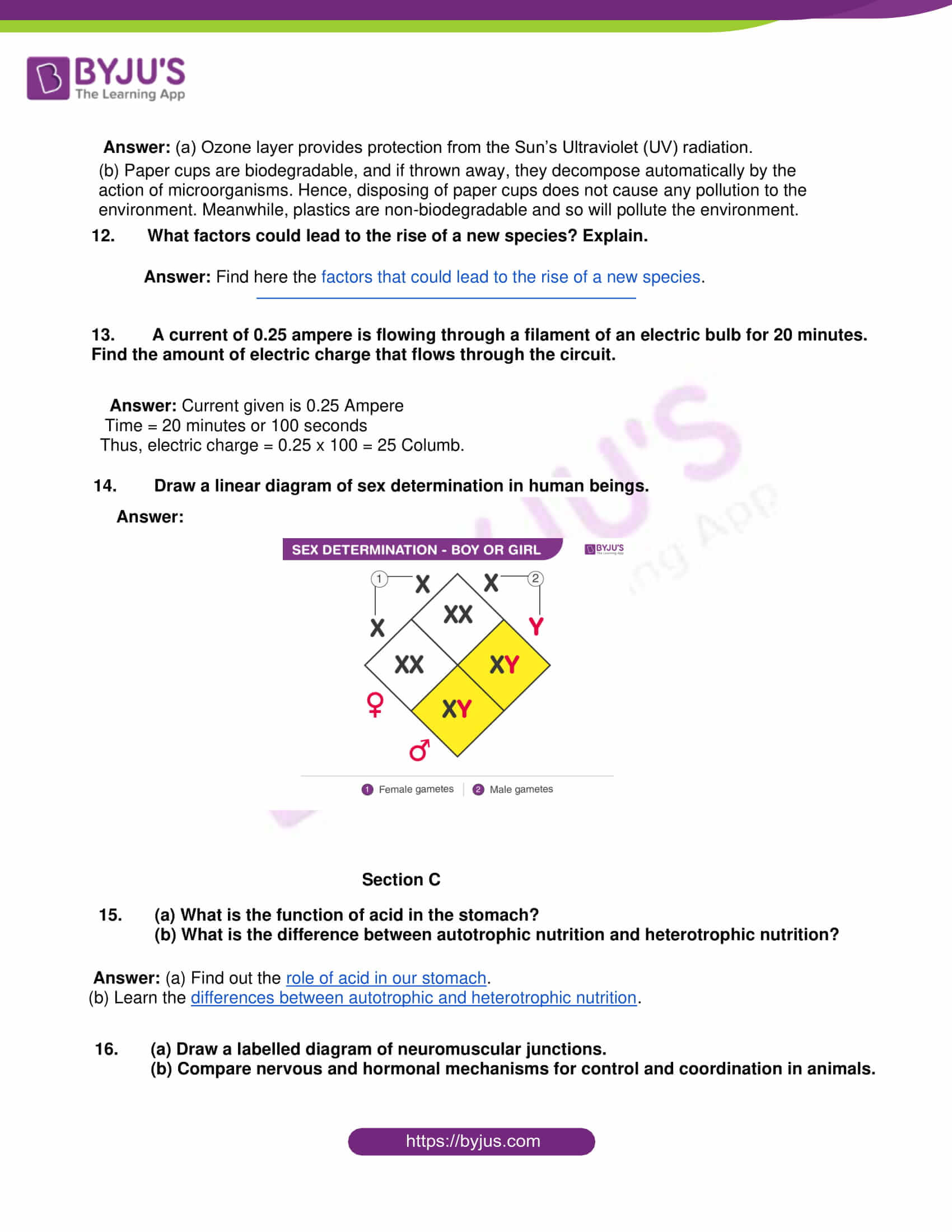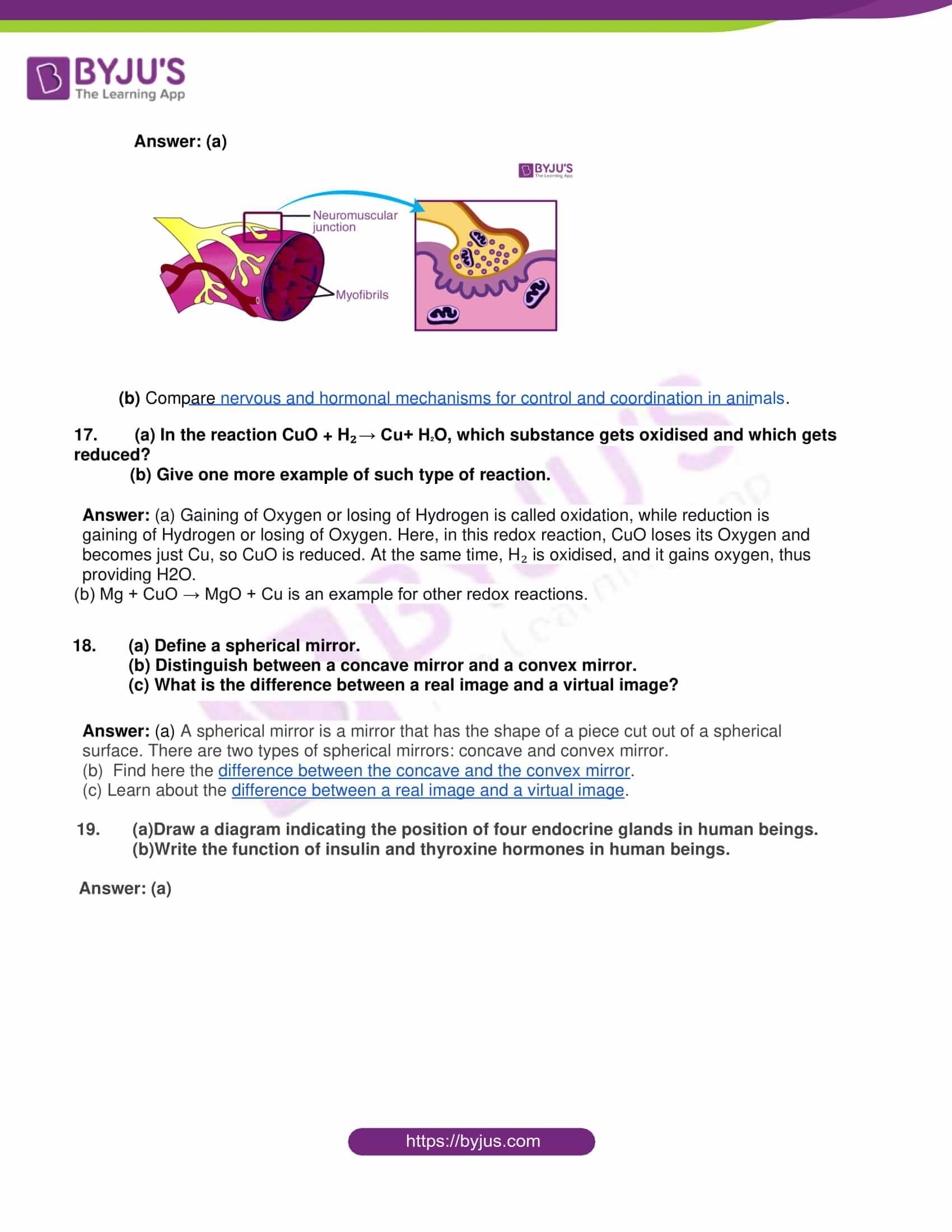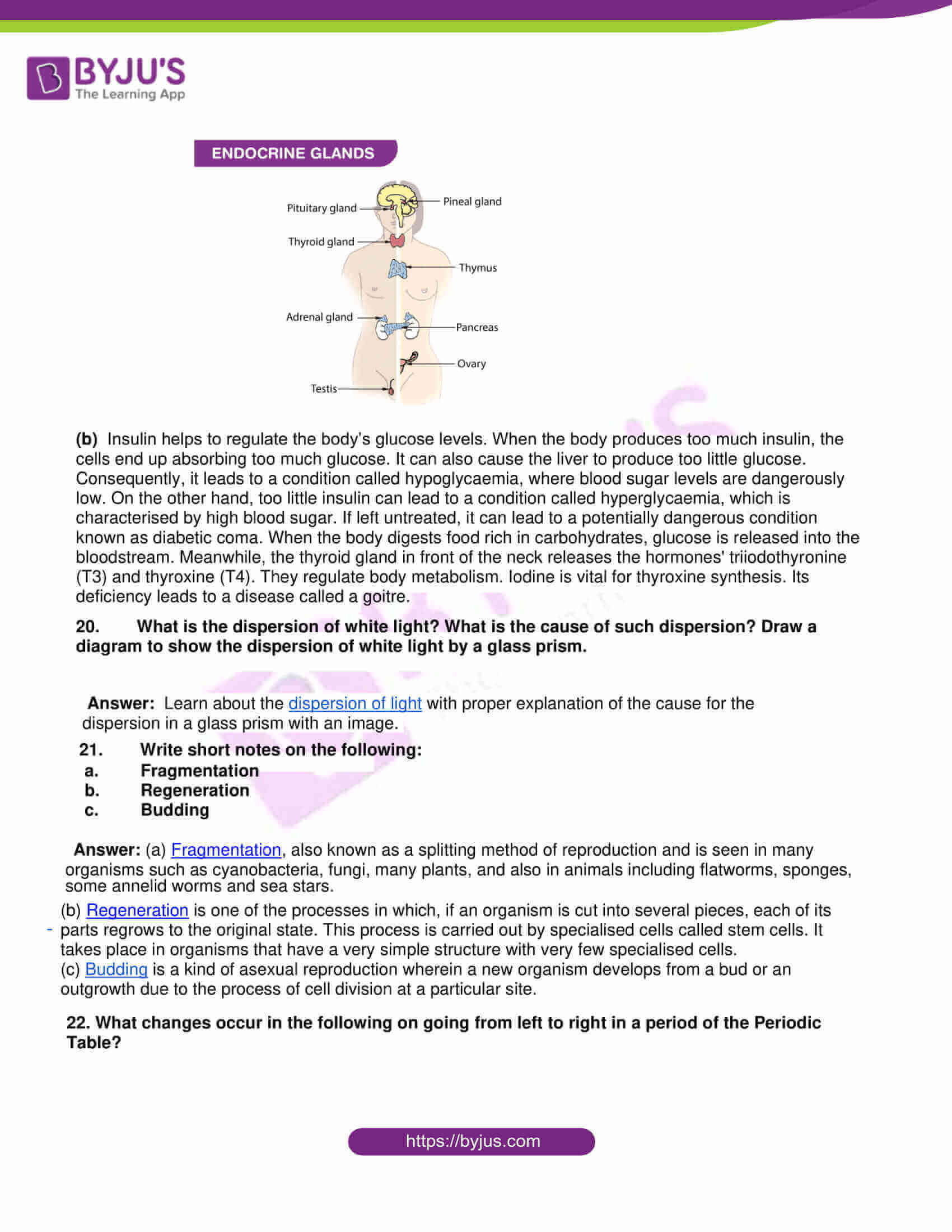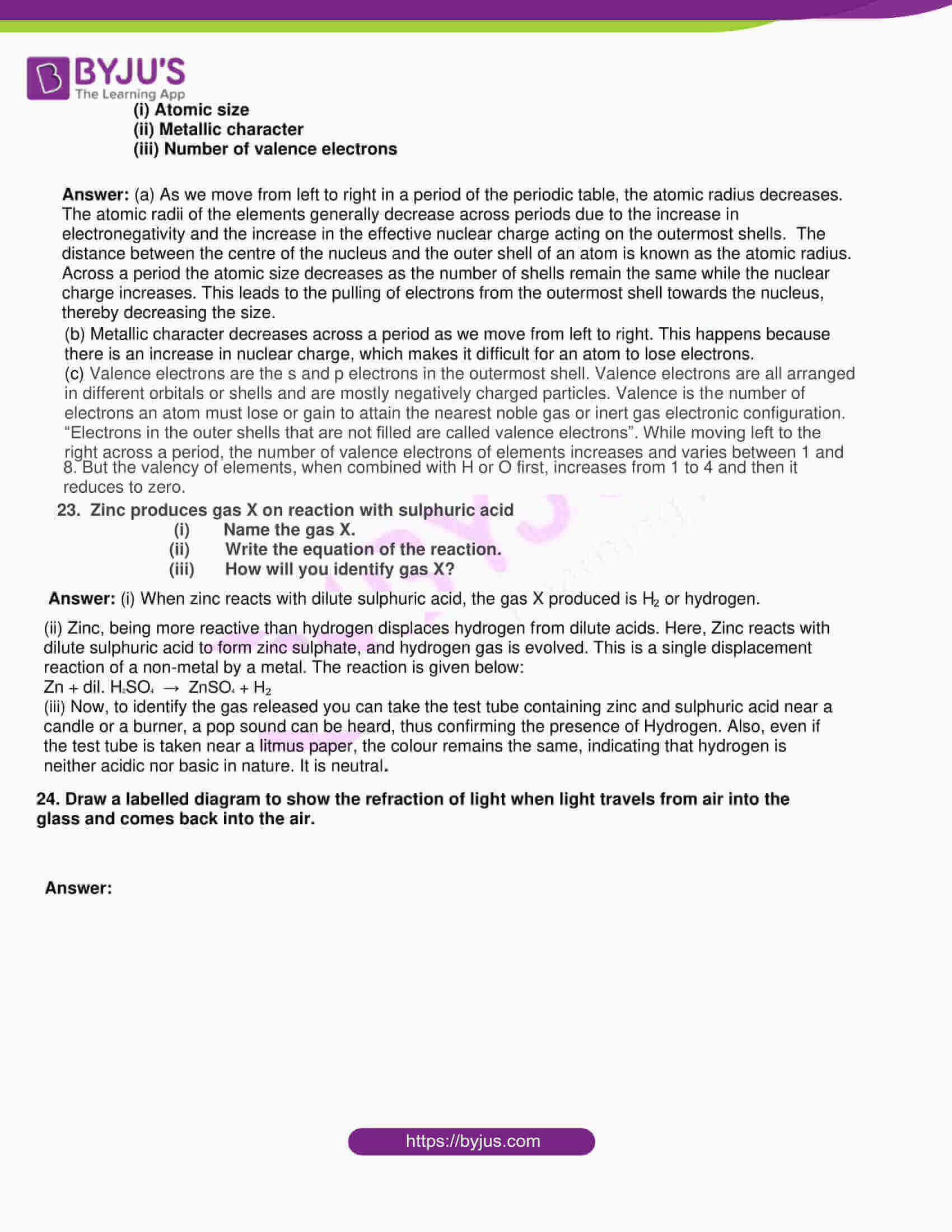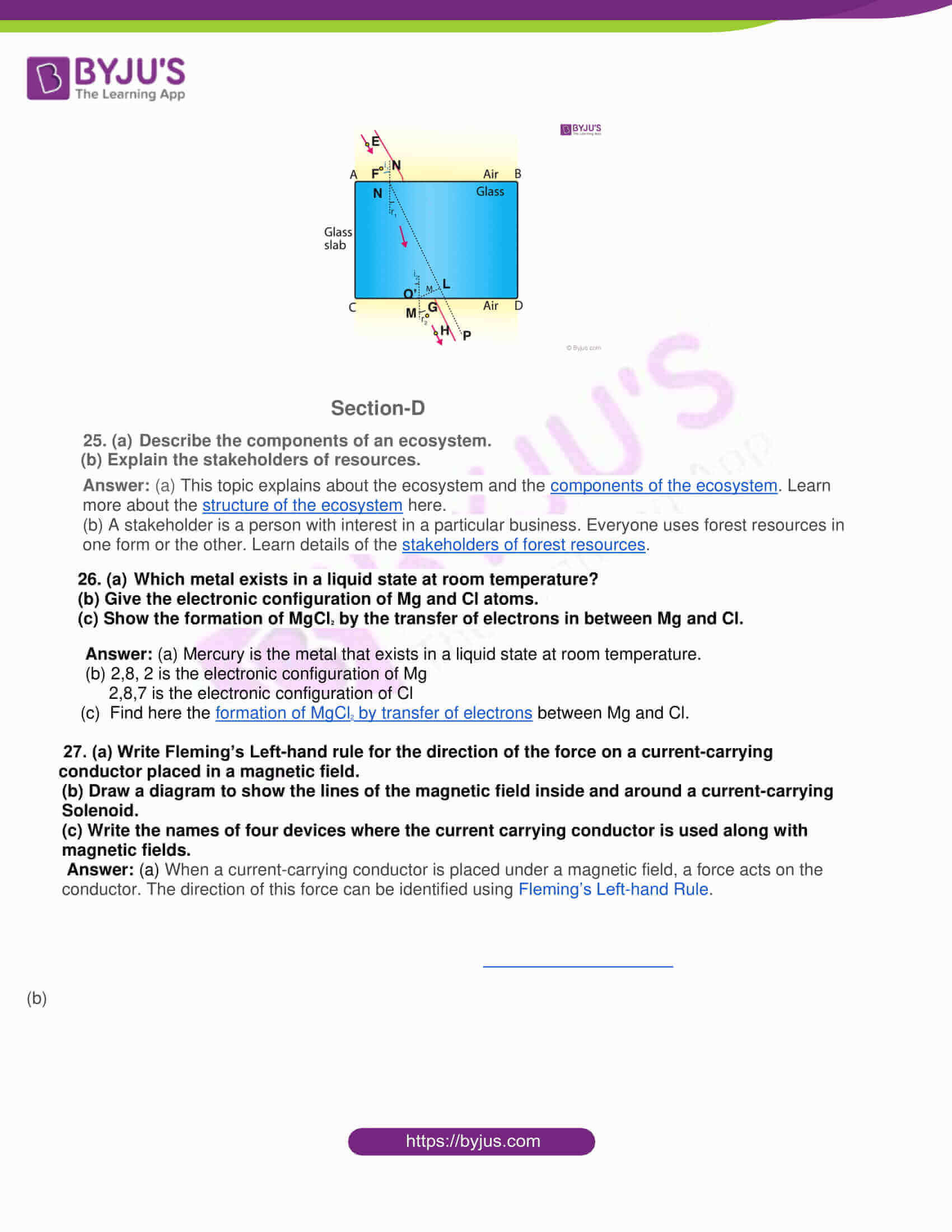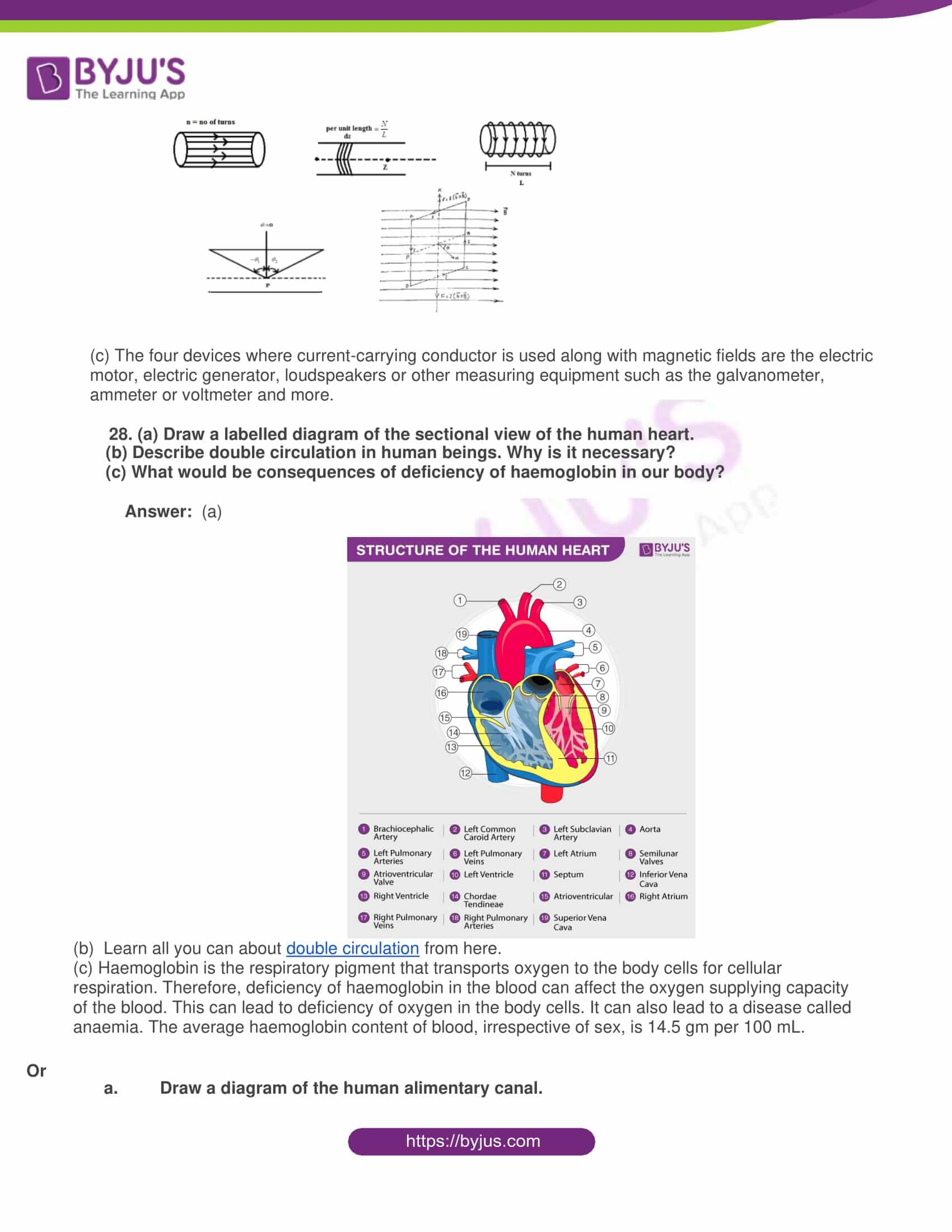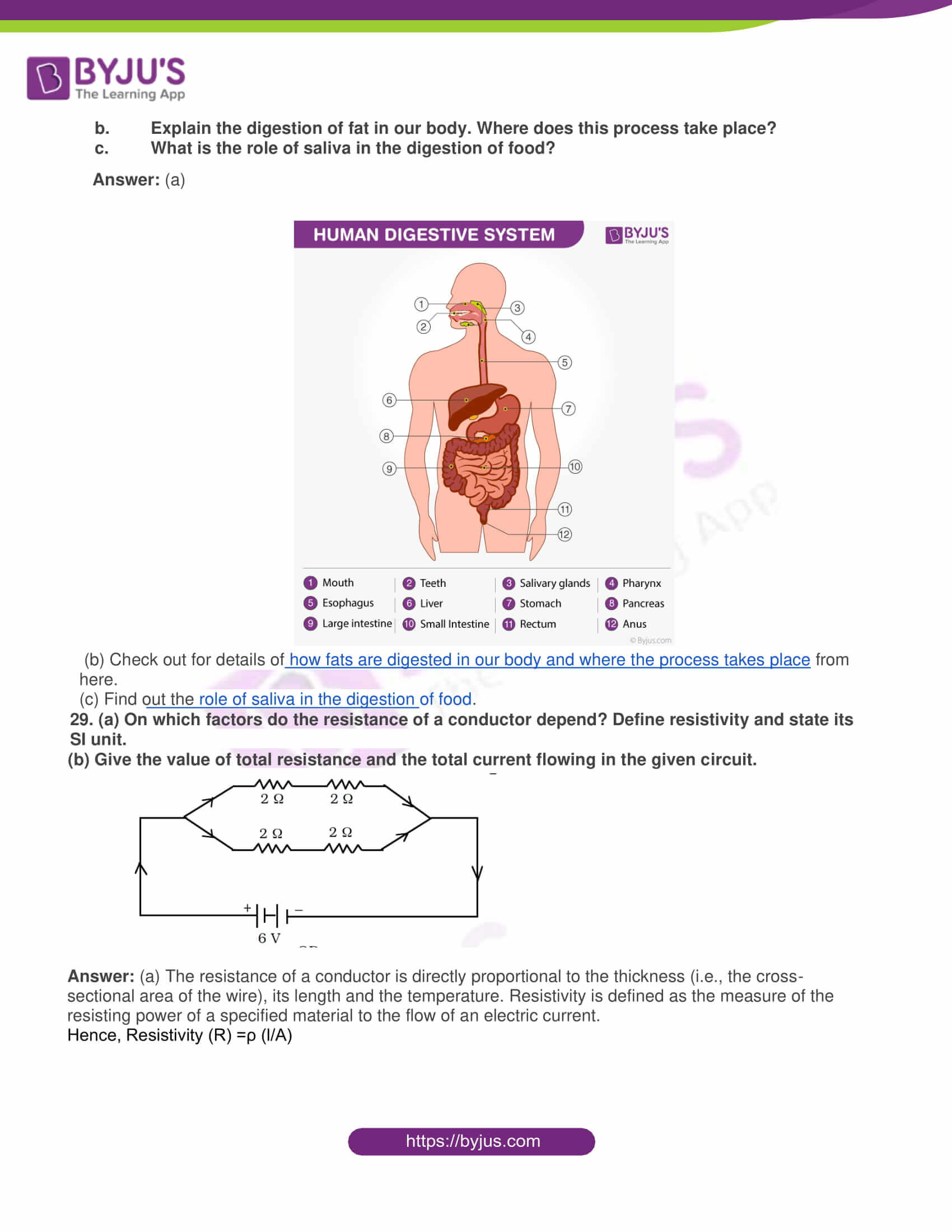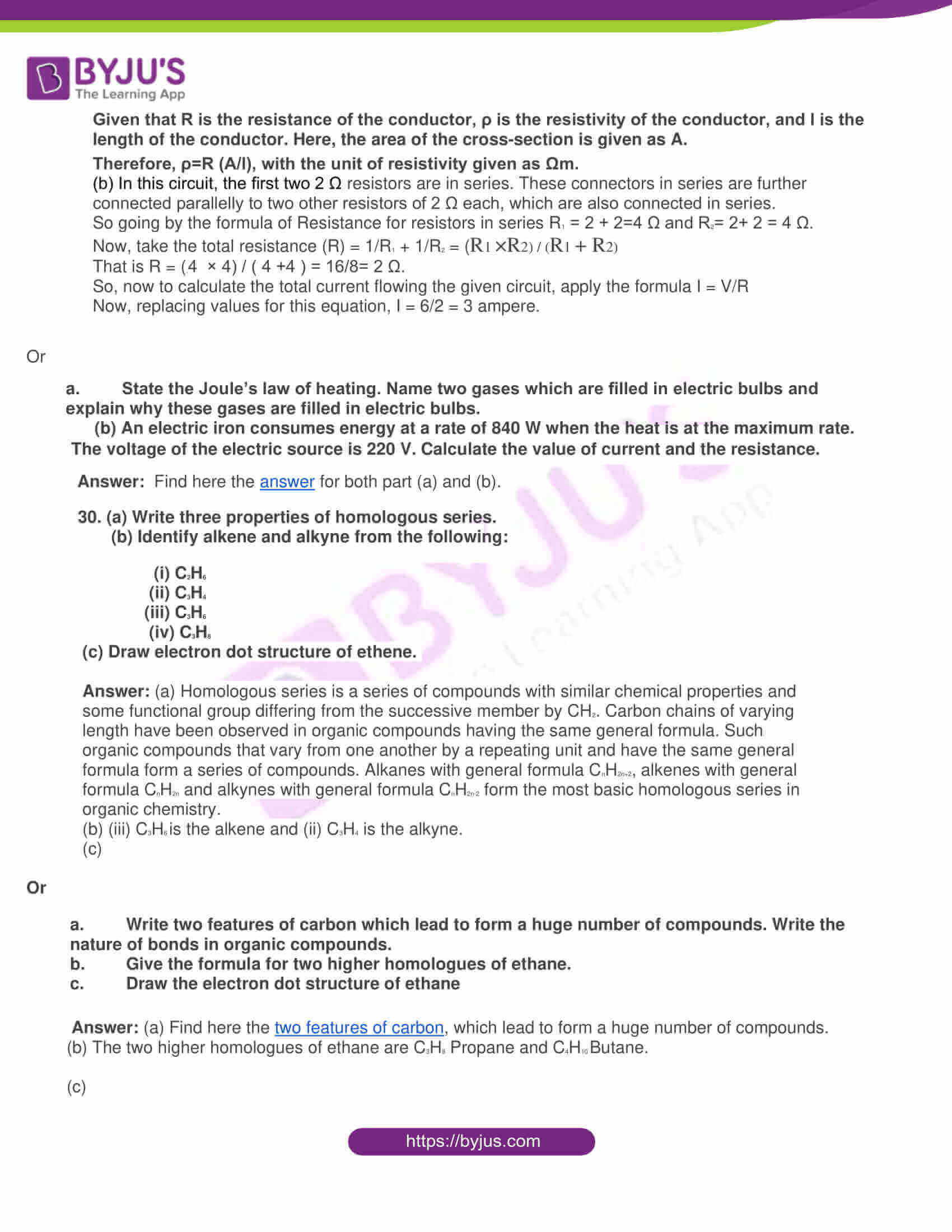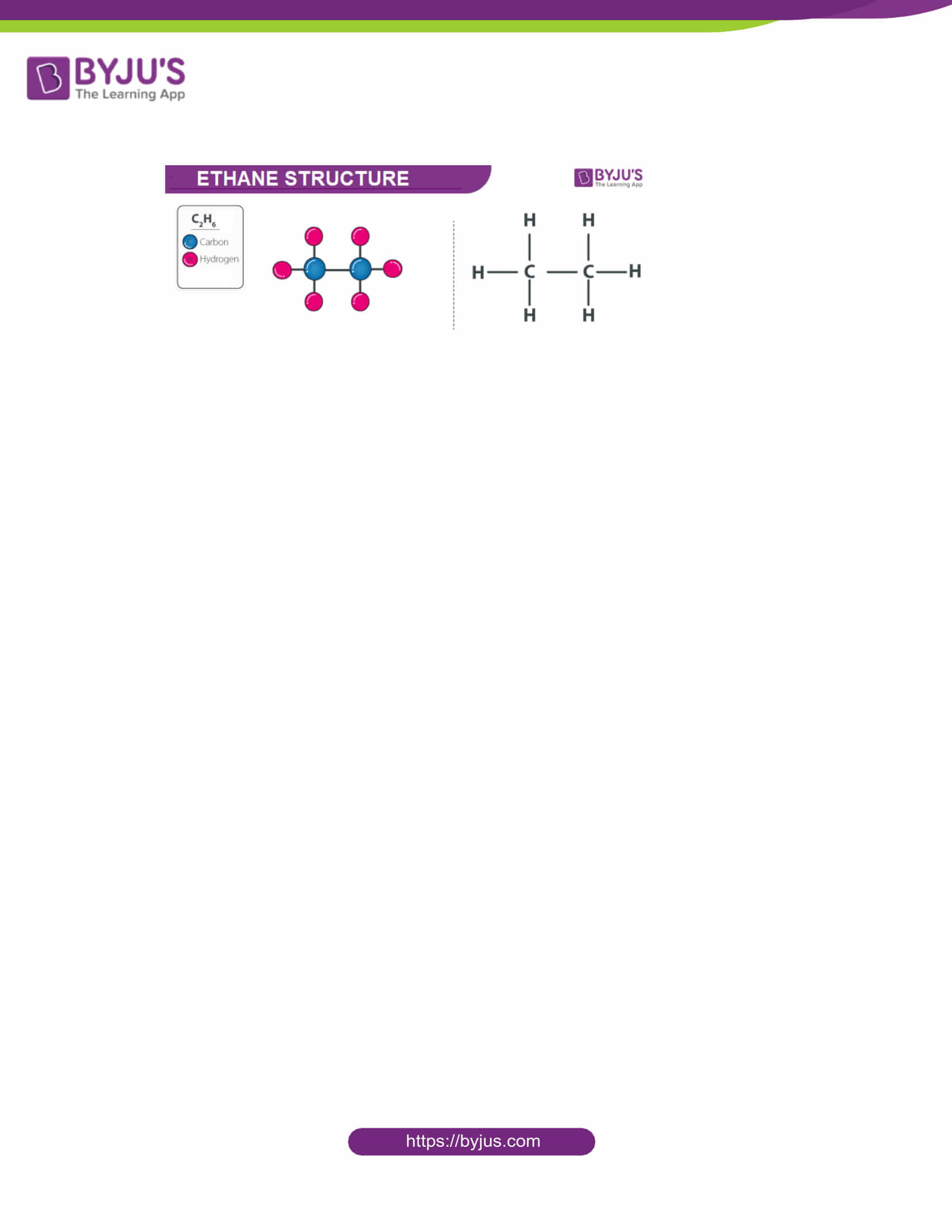Section- A

1. Name two synthetic indicators.

Answer: Indicators are the substances that give different colours to the medium to indicate if the solution is acidic or basic. Turmeric and beetroot are examples of natural indicators. Meanwhile, synthetic indicators are man-made and produced via synthetic processes. Phenolphthalein and methyl orange are examples of synthetic indicators.

2. Write the name of the structure, which provides nutrition to the embryo from the mother’s blood.

Answer: Placenta is a name of the structure that provides nutrition to the embryo from the mother’s blood. This organ develops in the uterus during pregnancy. Apart from providing nutrition to the developing baby, it also removes all the waste products from the baby’s blood.

3. Give the number of periods and groups in the Modern Periodic Table.

Answer: The periods of the table are the rows that go from left to right, while the elements of the group are from top to bottom. There are 7 periods and 18 groups in the Modern Periodic Tab.

4. Which one has a high pH value?

(i) Blood or distilled water

(ii) Gastric juice or lemon juice

Answer: (i) The pH value of blood is higher. Distilled water has a pH of 6.8 to 7.0, while the pH value of the blood is between 7.3 to 7.5.

(ii) Meanwhile, the pH of the gastric juice of the stomach is between 1.0 and 1.4, and that of lemon juice is higher at about 2.0.

5. What two precautions should be taken to avoid the overloading of domestic electric circuits?

6. What is the renewable and non-renewable source of energy?

Answer: The resources which cannot be exhausted even after continuous utilisation are termed as renewable resources. Examples of renewable resources are the sun, wind and tidal energy. The resources which cannot be immediately replaced once they are depleted are called Non-renewable resources. Examples of Non-renewable resources include fossil fuels, such as coal, petroleum and natural gas and rare minerals typically found in meteorites.

7. A domestic electric circuit has a fuse of 5A. What is the maximum number of 100 W (220 V) bulbs that can be safely used in the circuit?

Answer: Power of one bulb is 100 W, while the potential difference, (V) =22O V. Now, to find the current used by 1 bulb. Here, I is given as 5 Ampere. Imagine there are x bulbs

Power of x bulbs(P) = 100x watts

Apply the formula, P= VI

Therefore, replacing the values 100x = 220 × 5

x = (220 × 5) / 100 = 11 bulbs.

Thus, the maximum number of bulbs that can be safely used in the circuit is 11.’

8. What is the ability of the eye lens to adjust its focal length called?

Answer: Accommodation is known as the ability of the eye lens to adjust its focal length. Nevertheless, the focal length of the eye lens cannot be reduced below a certain minimum limit. If you try to read a printed page by holding it very close to your eyes, the image may become blurred, or you may feel strain in the eye. Hence, to see an object comfortably and distinctly, you must hold it at a distance of nearly 25 cm from the eyes and not any closer.

Section-B

9. Write the combination reaction and decomposition reaction with one example each.

Answer: In a combination reaction, two or more compounds or elements (reactants) combine to produce a single compound, that is the product. Example of a combination reaction is given here.

Ba + F2 ⟶ BaF2

The chemical reaction in which a single compound breaks down into new compounds or two or more elements is called a decomposition reaction. This reaction also involves some energy sources such as heat, light, electricity, which break apart the bonds of the compounds.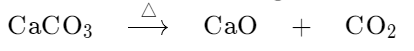10. (a) State the names of two elements that produce nuclear energy.

(b) What are high and low tides?

Answer: (a) Uranium and thorium are the two elements that produce nuclear energy.

(b) The gravitational forces of the sun and the moon combined with the rotation of the earth result in an alternate rise and fall of the sea levels. At one particular place, it usually occurs twice on a lunar day. The rise of the sea level is called the high tide, whereas the fall is called the low tide. When the earth and moon’s gravitational fields are in a straight line, the influences of these two fields become very strong and cause millions of gallons of the water flow towards the shore resulting in the high tide condition. Likewise, when the moon and earth’s gravitational fields are perpendicular to each other, the influences of these fields become weak, causing the water to flow away from the shore resulting in a low tide condition.

11. (a) Ozone layer provides protection from which radiation coming from the sun?

(b) What are the advantages of using disposable paper cups instead of disposable plastic cups?

Answer: (a) Ozone layer provides protection from the Sun’s Ultraviolet (UV) radiation.

(b) Paper cups are biodegradable, and if thrown away, they decompose automatically by the action of microorganisms. Hence, disposing of paper cups does not cause any pollution to the environment. Meanwhile, plastics are non-biodegradable and so will pollute the environment.

12. What factors could lead to the rise of a new species? Explain.

Answer: Find here the factors that could lead to the rise of a new species.

13. A current of 0.25 ampere is flowing through a filament of an electric bulb for 20 minutes. Find the amount of electric charge that flows through the circuit.

Answer: Current given is 0.25 Ampere

Time = 20 minutes or 100 seconds

Thus, electric charge = 0.25 x 100 = 25 Columb.

14. Draw a linear diagram of sex determination in human beings.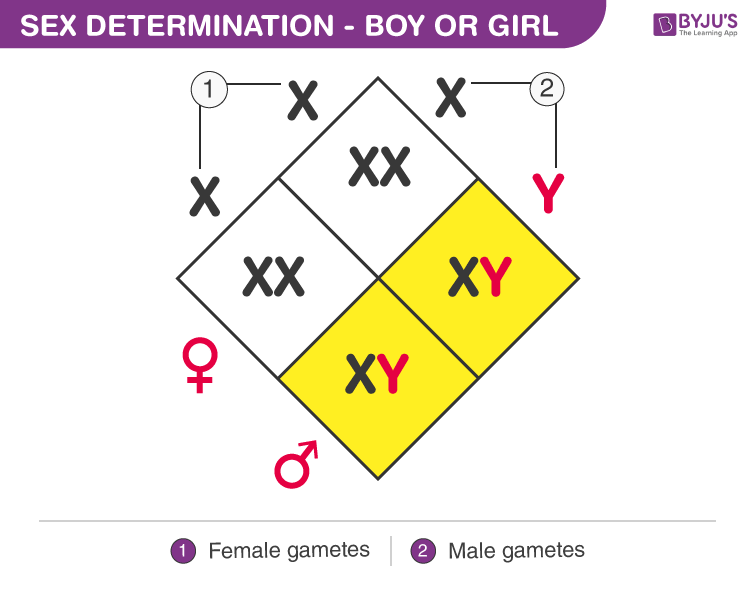Section C

15. (a) What is the function of acid in the stomach?

(b) What is the difference between autotrophic nutrition and heterotrophic nutrition?

Answer: (a) Find out the role of acid in our stomach.

16. (a) Draw a labelled diagram of neuromuscular junctions.

(b) Compare nervous and hormonal mechanisms for control and coordination in animals.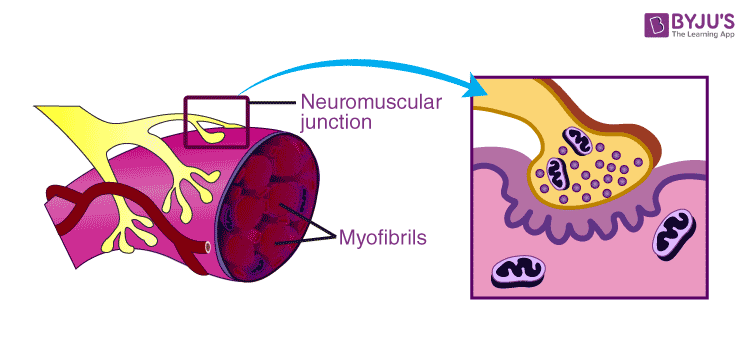17. (a) In the reaction CuO + H₂ → Cu+ H2O, which substance gets oxidised and which gets reduced?

(b) Give one more example of such type of reaction.

Answer: (a) Gaining of Oxygen or losing of Hydrogen is called oxidation, while reduction is gaining of Hydrogen or losing of Oxygen. Here, in this redox reaction, CuO loses its Oxygen and becomes just Cu, so CuO is reduced. At the same time, H₂ is oxidised, and it gains oxygen, thus providing H2O.

(b) Mg + CuO → MgO + Cu is an example for other redox reactions.

18. (a) Define a spherical mirror.

(b) Distinguish between a concave mirror and a convex mirror.

(c) What is the difference between a real image and a virtual image?

Answer: (a) A spherical mirror is a mirror that has the shape of a piece cut out of a spherical surface. There are two types of spherical mirrors: concave and convex mirror.

(b) Find here the difference between the concave and the convex mirror.

(c) Learn about the difference between a real image and a virtual image.

19. (a)Draw a diagram indicating the position of four endocrine glands in human beings.

(b)Write the function of insulin and thyroxine hormones in human beings.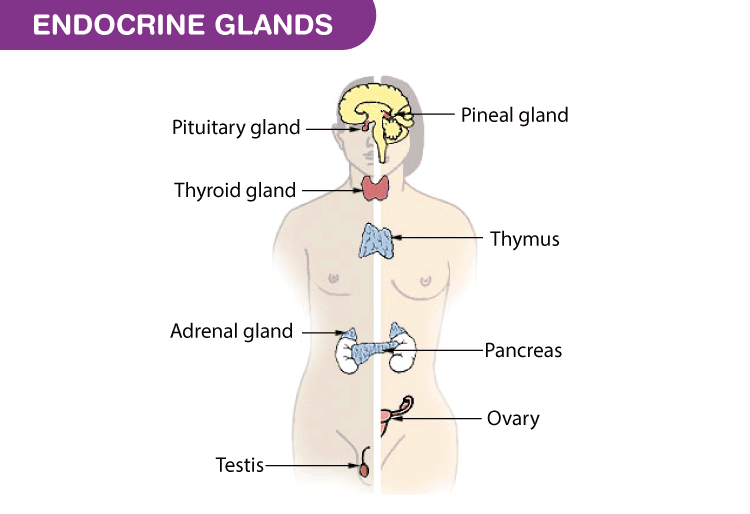(b) Insulin helps to regulate the body’s glucose levels. When the body produces too much insulin, the cells end up absorbing too much glucose. It can also cause the liver to produce too little glucose. Consequently, it leads to a condition called hypoglycaemia, where blood sugar levels are dangerously low. On the other hand, too little insulin can lead to a condition called hyperglycaemia, which is characterised by high blood sugar. If left untreated, it can lead to a potentially dangerous condition known as diabetic coma. When the body digests food rich in carbohydrates, glucose is released into the bloodstream. Meanwhile, the thyroid gland in front of the neck releases the hormones’ triiodothyronine (T3) and thyroxine (T4). They regulate body metabolism. Iodine is vital for thyroxine synthesis. Its deficiency leads to a disease called a goitre.

20. What is the dispersion of white light? What is the cause of such dispersion? Draw a diagram to show the dispersion of white light by a glass prism.

Answer: Learn about the dispersion of light with proper explanation of the cause for the dispersion in a glass prism with an image.

1. Write short notes on the following:
2. Fragmentation
3. Regeneration
4. Budding

Answer: (a) Fragmentation, also known as a splitting method of reproduction and is seen in many organisms such as cyanobacteria, fungi, many plants, and also in animals including flatworms, sponges, some annelid worms and sea stars.

(b)
Regeneration is one of the processes in which, if an organism is cut into several pieces, each of its parts regrows to the original state. This process is carried out by specialised cells called stem cells. It takes place in organisms that have a very simple structure with very few specialised cells.

(c) Budding is a kind of asexual reproduction wherein a new organism develops from a bud or an outgrowth due to the process of cell division at a particular site.

22. What changes occur in the following on going from left to right in a period of the Periodic Table?

(i) Atomic size

(ii) Metallic character

(iii) Number of valence electrons

Answer: (a) As we move from left to right in a period of the periodic table, the atomic radius decreases. The atomic radii of the elements generally decrease across periods due to the increase in electronegativity and the increase in the effective nuclear charge acting on the outermost shells. The distance between the centre of the nucleus and the outer shell of an atom is known as the atomic radius. Across a period the atomic size decreases as the number of shells remain the same while the nuclear charge increases. This leads to the pulling of electrons from the outermost shell towards the nucleus, thereby decreasing the size.

(b) Metallic character decreases across a period as we move from left to right. This happens because there is an increase in nuclear charge, which makes it difficult for an atom to lose electrons.

(c) Valence electrons are the s and p electrons in the outermost shell. Valence electrons are all arranged in different orbitals or shells and are mostly negatively charged particles. Valence is the number of electrons an atom must lose or gain to attain the nearest noble gas or inert gas electronic configuration. “Electrons in the outer shells that are not filled are called valence electrons”. While moving left to the right across a period, the number of valence electrons of elements increases and varies between 1 and 8. But the valency of elements, when combined with H or O first, increases from 1 to 4 and then it reduces to zero.

23. Zinc produces gas X on reaction with sulphuric acid

(i) Name the gas X.

(ii) Write the equation of the reaction.

(iii) How will you identify gas X?

Answer: (i) When zinc reacts with dilute sulphuric acid, the gas X produced is H₂ or hydrogen.

(ii) Zinc, being more reactive than hydrogen displaces hydrogen from dilute acids. Here, Zinc reacts with dilute sulphuric acid to form zinc sulphate, and hydrogen gas is evolved. This is a single displacement reaction of a non-metal by a metal. The reaction is given below:

Zn + dil. H2SO4 → ZnSO4 + H₂

(iii) Now, to identify the gas released you can take the test tube containing zinc and sulphuric acid near a candle or a burner, a pop sound can be heard, thus confirming the presence of Hydrogen. Also, even if the test tube is taken near a litmus paper, the colour remains the same, indicating that hydrogen is neither acidic nor basic in nature. It is neutral.

24. Draw a labelled diagram to show the refraction of light when light travels from air into the glass and comes back into the air.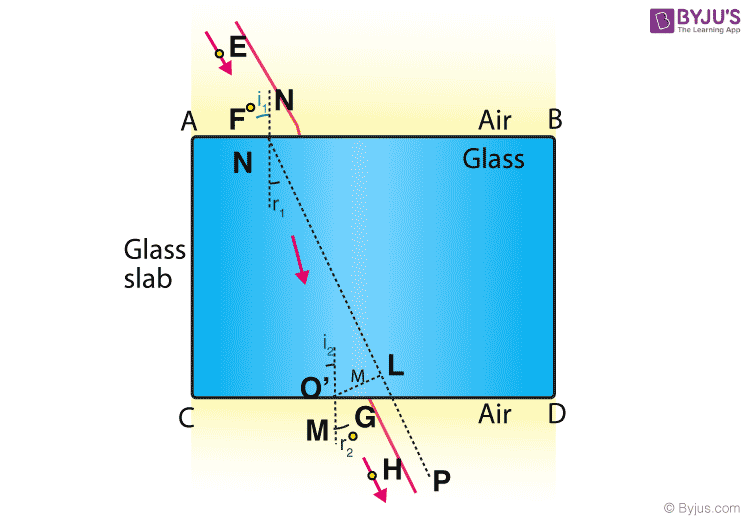Section-D

25. (a) Describe the components of an ecosystem.

(b) Explain the stakeholders of resources.

(b) A stakeholder is a person with interest in a particular business. Everyone uses forest resources in one form or the other. Learn details of the stakeholders of forest resources.

26. (a) Which metal exists in a liquid state at room temperature?

(b) Give the electronic configuration of Mg and Cl atoms.

(c) Show the formation of MgCl2 by the transfer of electrons in between Mg and Cl.

Answer: (a) Mercury is the metal that exists in a liquid state at room temperature.

(b) 2,8, 2 is the electronic configuration of Mg

2,8,7 is the electronic configuration of Cl

(c) Find here the formation of MgCl2 by transfer of electrons between Mg and Cl.

27. (a) Write Fleming’s Left-hand rule for the direction of the force on a current-carrying conductor placed in a magnetic field.

(b) Draw a diagram to show the lines of the magnetic field inside and around a current-carrying Solenoid.

(c) Write the names of four devices where the current carrying conductor is used along with magnetic fields.

Answer: (a) When a current-carrying conductor is placed under a magnetic field, a force acts on the conductor. The direction of this force can be identified using Fleming’s Left-hand Rule.

(b)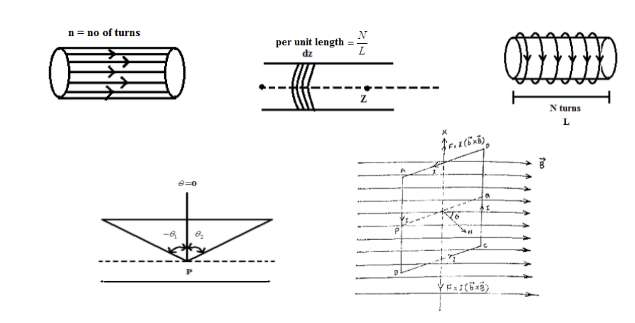(c) The four devices where current-carrying conductor is used along with magnetic fields are the electric motor, electric generator, loudspeakers or other measuring equipment such as the galvanometer, ammeter or voltmeter and more.

28. (a) Draw a labelled diagram of the sectional view of the human heart.

(b) Describe double circulation in human beings. Why is it necessary?

(c) What would be consequences of deficiency of haemoglobin in our body?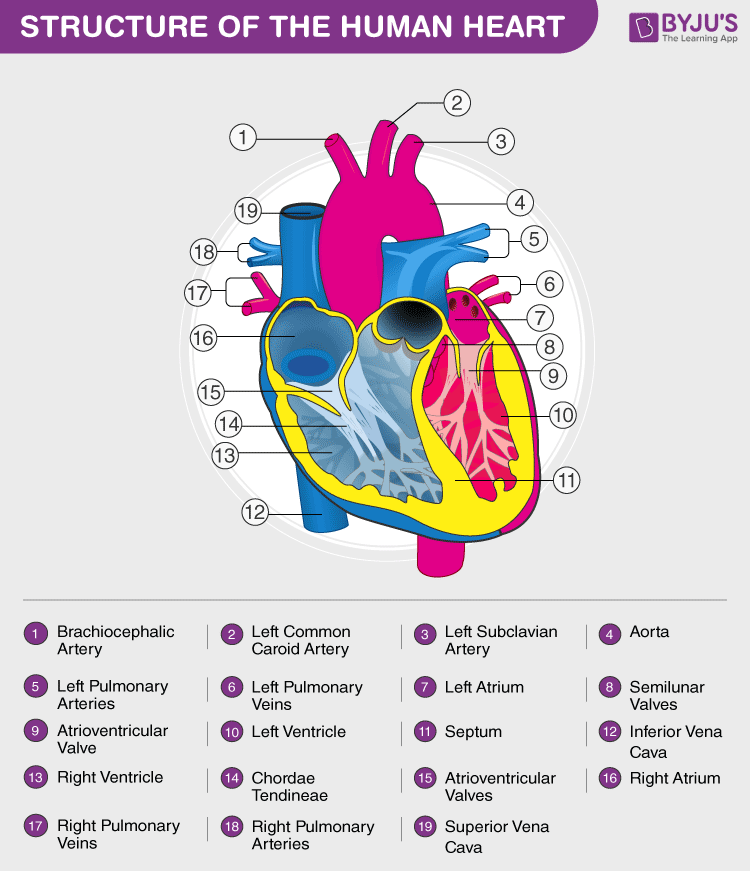(b) Learn all you can about double circulation from here.

(c) Haemoglobin is the respiratory pigment that transports oxygen to the body cells for cellular respiration. Therefore, deficiency of haemoglobin in the blood can affect the oxygen supplying capacity of the blood. This can lead to deficiency of oxygen in the body cells. It can also lead to a disease called anaemia. The average haemoglobin content of blood, irrespective of sex, is 14.5 gm per 100 mL.

Or

1. Draw a diagram of the human alimentary canal.
2. Explain the digestion of fat in our body. Where does this process take place?
3. What is the role of saliva in the digestion of food?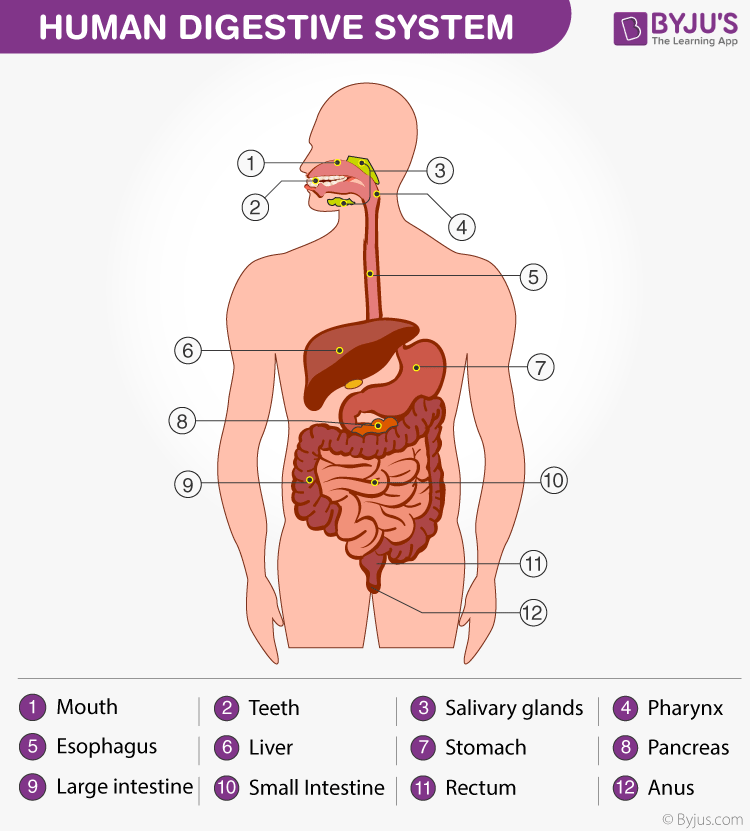(b) Check out for details of how fats are digested in our body and where the process takes place from here.

(c) Find out the role of saliva in the digestion of food.

29. (a) On which factors do the resistance of a conductor depend? Define resistivity and state its SI unit.

(b) Give the value of total resistance and the total current flowing in the given circuit.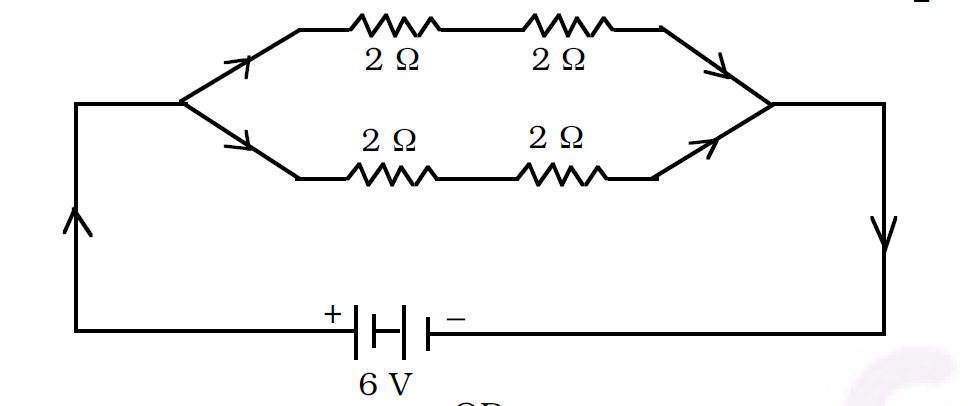Answer: (a) The resistance of a conductor is directly proportional to the thickness (i.e., the cross-sectional area of the wire), its length and the temperature. Resistivity is defined as the measure of the resisting power of a specified material to the flow of an electric current.

Hence, Resistivity (R) =ρ (l/A) ​

Given that R is the resistance of the conductor, ρ is the resistivity of the conductor, and l is the length of the conductor. Here, the area of the cross-section is given as A.

Therefore, ρ=R (A/l), with the unit of resistivity given as Ωm.

(b) In this circuit, the first two 2 Ω resistors are in series. These connectors in series are further connected parallelly to two other resistors of 2 Ω each, which are also connected in series.

So going by the formula of Resistance for resistors in series R1 = 2 + 2=4 Ω and R2= 2+ 2 = 4 Ω.

Now, take the total resistance (R) = 1/R1 + 1/R2 = (R1 ×R2) / (R1 + R2)

That is R = (,4 × 4) / ( 4 +4 ) = 16/8= 2 Ω.

So, now to calculate the total current flowing the given circuit, apply the formula I = V/R

Now, replacing values for this equation, I = 6/2 = 3 ampere.

Or

30. State the Joule’s law of heating. Name two gases which are filled in electric bulbs and explain why these gases are filled in electric bulbs.

(b) An electric iron consumes energy at a rate of 840 W when the heat is at the maximum rate. The voltage of the electric source is 220 V. Calculate the value of current and the resistance.

30. (a) Write three properties of homologous series.

(b) Identify alkene and alkyne from the following:

(i) C2H6

(ii) C3H4

(iii) C3H6

(iv) C3H8

(c) Draw electron dot structure of ethene.

Answer: (a) Homologous series is a series of compounds with similar chemical properties and some functional group differing from the successive member by CH2. Carbon chains of varying length have been observed in organic compounds having the same general formula. Such organic compounds that vary from one another by a repeating unit and have the same general formula form a series of compounds. Alkanes with general formula CnH2n+2, alkenes with general formula CnH2n and alkynes with general formula CnH2n-2 form the most basic homologous series in organic chemistry.

(b) (iii) C3H6 is the alkene and (ii) C3H4 is the alkyne.

(c)

Or

1. Write two features of carbon which lead to form a huge number of compounds. Write the nature of bonds in organic compounds.
2. Give the formula for two higher homologues of ethane.
3. Draw the electron dot structure of ethane

Answer: (a) Find here the two features of carbon, which lead to form a huge number of compounds.

(b) The two higher homologues of ethane are C3H8 Propane and C4H10 Butane.

(c)